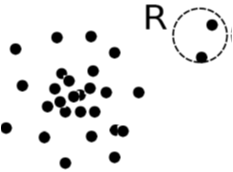# Current Slide

Small screen detected. You are viewing the mobile version of SlideWiki. If you wish to edit slides you will need to use a larger device.

### Outlier Detection (1): Statistical Methods

• Statistical methods (also known as model-based methods) assume that the normal data follow some statistical model (a stochastic model)
• The data not following the model are outliers.
• Effectiveness of statistical methods: highly depends on whether the assumption of statistical model holds in the real data
• There are rich alternatives to use various statistical models
• E.g., parametric vs. non-parametric
• Example (below figure): First use Gaussian distribution to model the normal data
• For each object y in region R, estimate gD(y), the probability of y fits the Gaussian distribution
• If gD(y) is very low, y is unlikely generated by the Gaussian model, thus an outlierSpeaker notes:

## Content Tools

### Sources

There are currently no sources for this slide.